PROC HPSUMMARY Statement

Subsections:

PROC HPSUMMARY <options> <statistic-keywords> ;

The PROC HPSUMMARY statement invokes the procedure. The HPSUMMARY procedure computes descriptive statistics for variables across all observations or within groups of observations.

Table 10.1 summarizes the available options in the PROC HPSUMMARY statement by function. The options are then described fully in alphabetical order in the section Optional Arguments. For information about the statistic-keywords, see the section Statistic Keywords.

Table 10.1: PROC HPSUMMARY Statement Options

Option

Description

Basic Options

Specifies the input data set

Specifies the mathematical definition used to compute quantiles

Option Related to Classification Level

Uses missing values as valid values to create combinations of classification variables

Options Related to the Output Data Set

Computes statistics for all combinations of classification variables (not just the-way)

Specifies that the _TYPE_ variable contain character values

Options Related to Statistical Analysis

Specifies the confidence level for the confidence limits

Excludes observations with nonpositive weights from the analysis

Specifies the sample size to use for the P2 quantile estimation method

Specifies the quantile estimation method

Specifies the mathematical definition used to compute quantiles

Selects the statistics

Specifies the variance divisor

Optional Arguments

You can specify the following options in the PROC HPSUMMARY statement:

ALLTYPES
ALLWAYS

requests that PROC HPSUMMARY compute descriptive statistics for all combinations of classification variables. By default, PROC HPSUMMARY generates only the-way. For more information, see the section How PROC HPSUMMARY Groups Data.

ALPHA= value

specifies the confidence level to compute the confidence limits for the mean. The percentage for the confidence limits is 100(1–value). For example, ALPHA=0.05 results in a 95% confidence limit. You can specify any value between 0 and 1. The default is 0.05. To compute confidence limits, specify the statistic-keyword CLM, LCLM, or UCLM. See the section Confidence Limits.

CHARTYPE

specifies that the _TYPE_ variable in the output data set is a character representation of the binary value of _TYPE_. The length of the variable equals the number of classification variables. When you specify more than 32 classification variables, _TYPE_ automatically becomes a character variable. See the section Output Data Set.

DATA= SAS-data-set

names the SAS data set to be used as the input data set. The default is the most recently created data set.

EXCLNPWGT
EXCLNPWGTS

excludes observations with nonpositive weight values (0 or negative) from the analysis. By default, PROC HPSUMMARY treats observations with negative weights like observations with zero weights and counts them in the total number of observations. See the WEIGHT= option and the section WEIGHT Statement.

MISSING

considers missing values as valid values to create the combinations of classification variables. Special missing values that represent numeric values—the letters A through Z and the underscore (_) character—are each considered as a separate value. If you omit MISSING, then PROC HPSUMMARY excludes the observations with a missing classification variable value from the analysis. See SAS Language Reference: Concepts for a discussion of missing values that have special meanings.

PCTLDEF=1 | 2 |  3 | 4 |  5

is an alias for the QNTLDEF= option.

QMARKERS= number

specifies the default number of markers to use for the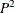quantile estimation method. The number of markers controls the size of fixed memory space.

The value of number must be an odd integer greater than 3. The default value depends on which quantiles you request. For the median (P50), number is 7. For the quantiles (P25 and P50), number is 25. For the quantiles P1, P5, P10, P75 P90, P95, or P99, number is 105. If you request several quantiles, then PROC HPSUMMARY uses the largest value of number.

You can improve the accuracy of the estimate by increasing the number of markers above the default settings; you can conserve memory and computing time by reducing the number of markers. See the section Quantiles.

QMETHOD=OS | P2

specifies the method that PROC HPSUMMARY uses to process the input data when it computes quantiles. If the number of observations is less than or equal to the QMARKERS= value and QNTLDEF=5, then both methods produce the same results. The QMETHOD= option can take either of the following values:

OS

specifies that PROC HPSUMMARY use order statistics.

Note: This technique can be very memory-intensive.

P2

specifies that PROC HPSUMMARY use themethod to approximate the quantile. When QMETHOD=P2, PROC HPSUMMARY does not compute MODE or weighted quantiles. In addition, reliable estimations of some quantiles (P1, P5, P95, P99) might not be possible for some data sets.

QNTLDEF=1 | 2 |  3 | 4 |  5
PCTLDEF=1 | 2 |  3 | 4 |  5

specifies the mathematical definition that PROC HPSUMMARY uses to calculate quantiles when QMETHOD=OS. The default is 5. To use QMETHOD=P2, you must use QNTLDEF=5. See the section Quantile and Related Statistics.

VARDEF= divisor

specifies the divisor to use in the calculation of the variance and standard deviation. Table 10.2 shows the possible values for divisor and their associated formulas.

Table 10.2: Values for VARDEF= Option

divisor

Description

Formula for Divisor

DF

Degrees of freedom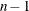N

Number of observationsWDF

Sum of weights minus one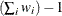WEIGHT | WGT

Sum of weights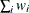The procedure computes the variance as CSS divided by divisor, where the corrected sum of squares CSS is defined by the following formula: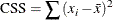When you weight the analysis variables, the formula for CSS is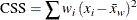where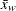is the weighted mean.

The default is DF. To compute the standard error of the mean, confidence limits for the mean, or the Student’s-test, you must use this default value.

When you use the WEIGHT statement and VARDEF=DF, the variance is an estimate of, where the variance of theth observation is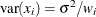and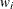is the weight for theth observation. This method yields an estimate of the variance of an observation with unit weight. When you use the WEIGHT statement and VARDEF=WGT, the computed variance is asymptotically (for large) an estimate of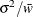, where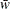is the average weight. This method yields an asymptotic estimate of the variance of an observation with average weight. See the section Keywords and Formulas.